Chapter Notes: Surface Area & Volumes

# Surface Area & Volumes Chapter Notes - Mathematics (Maths) Class 9

## Introduction to Area and Volume

The shapes that we have studied in previous classes like rectangles, squares, triangle, and circles are the plane figures which can be easily drawn on our notebooks. Suppose, we cut out a circle shape from a cardboard sheet. And then we cut many such circles identical to the first one and then pile them up in a single column. [Shown in the figure] Then the shape we obtained will be a 3-dimensional shape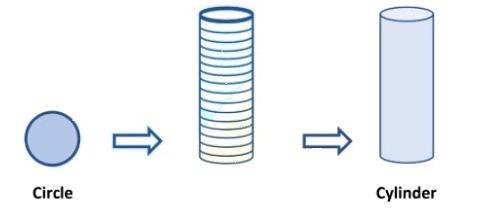By this process, we shall obtain some solid figures such as a cuboid and cube as shown below.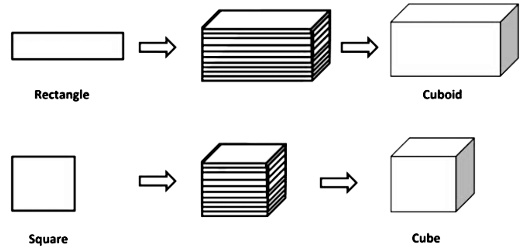All solid objects occupy some space and have three dimensions - length, breadth, and height or depth. Three dimensional (3-d)shapes have four parts that set them apart from 2-d shapes viz. faces, vertices, edges, and volume.
Some real-life examples which resemble solid shapes as shown below.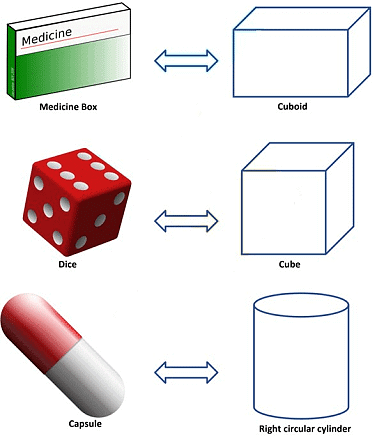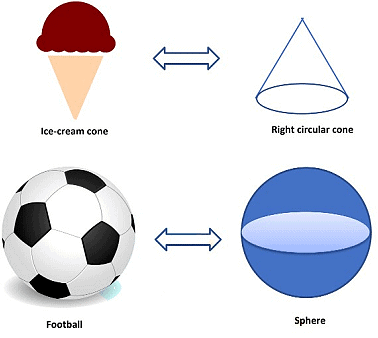### Surface Area of a Cuboid

Cuboid: In our day to day life, we come across various objects which are in the shape of a cuboid. Some examples are shown below.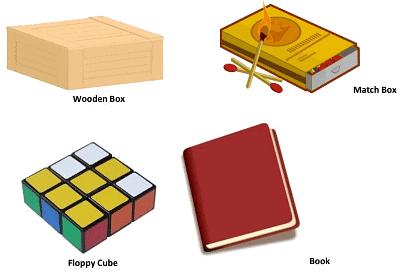If we observe closely, we find that all these objects are made up of six rectangular plane regions. A cuboid is a three-dimensional shape that has length, width, and height. The cuboid shape has six surfaces called faces. Each face of a cuboid is a rectangle, two adjacent faces of a cuboid meet in a line segment is called edge and all of a cuboid's corners (called vertices) are right angles.

Ultimately, a cuboid has the shape of a rectangular box.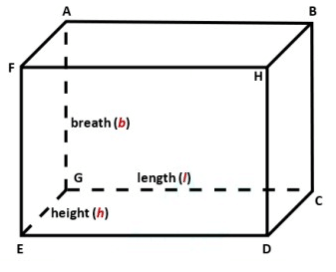A cuboid has sixfaces (FHDE, HBCD, CDEG, GEFA, FABH & ABCG), eightvertices (A, B, C, D, E, F, G & H), twelve edges (AB, BH, FH, AF, BC, CD, DH, DE, FE & GC).
If we want to cover cuboid by gift paper, first we would need a rectangular piece to cover the bottom of the cuboid.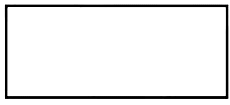Then we would need two long rectangular pieces to cover the two side ends. Now, it would look like as shown below.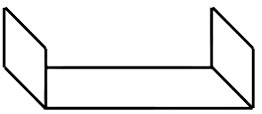Now to cover the front and back end, we would need two more rectangle pieces of the same size as them, we get a figure as shown below.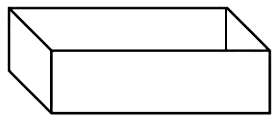This figure, when opened out, would look like as shown below.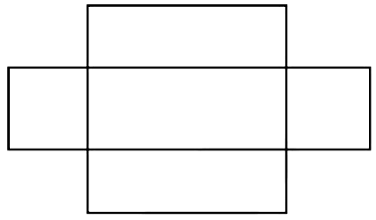Finally, to cover the top of the cuboid, we would require another rectangular piece exactly like the one at the bottom, which if we attached on the right side, it would look like as shown below.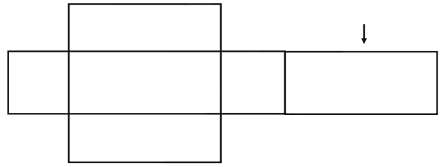Therefore, we have used six rectangular pieces to cover the complete outer surface of the cuboid.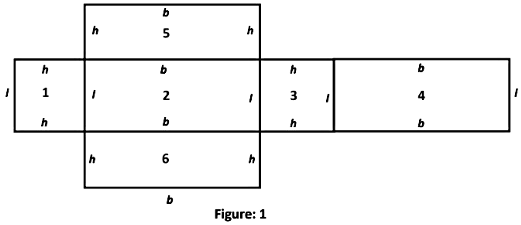This shows us that the outer surface of a cuboid is made up of six rectangular surfaces, called faces of the cuboid, whose area can be formed by multiplying the length by breath for each of them separately and then adding the six areas together.
Now, if we take the length of the cuboid as l, breadth as b and the height as h, then the figure with these dimensions would be like the shape shown in the figure: 1.
So, the sum of the areas of the six rectangles is:
Area of rectangle 1 = (l × h) + Area of rectangle 2 = (l × b) +
Area of rectangle 3 = (l × h) + Area of rectangle 4 = (l × b) +
Area of rectangle 5 = (b × h) + Area of rectangle 6 = (b × h)
⇒ Surface Area of Cuboid = 2(l × b) + 2(b × h) + 2(l × h).
= 2[(l × b) + (b × h) + (l × h)].
= 2(lb + bh + lh).
Where l, b and h are respectively the three edges of the cuboid.
Surface Area of Cuboid = 2(lb + bh + lh)

Note: Surface Area of Cuboid is also known as the Total Surface Area of Cuboid.

Example: Find the surface area of a wooden box whose length, breadth and height are 10 cm, 8 cm, and 6 cm, respectively.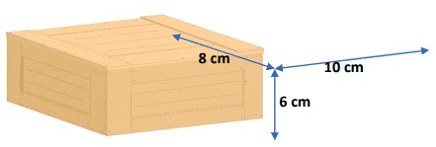Clearly, this wooden box is in the form of a cuboid.
Here, l = 10 cm, b = 8 cm and h = 6 cm.
Therefore, surface area of the wooden box = 2(lb + bh + lh).
= 2[(10 cm × 8 cm) + (8 cm × 6 cm) + (10 cm × 6 cm)].
= 2(80 cm2 + 48 cm2+ 60 cm2).
= 2× 188cm2.
= 376 cm2.
Hence, the surface area of the wooden box =  376 cm2.

Lateral Surface Area of a Cuboid: If out of the six faces of a cuboid, we only find the sum of the areas of four faces leaving the bottom and top faces. This sum is called the lateral surface area of a cuboid.
Consider a cuboid of length l, breadth b and height h as shown in the figure below.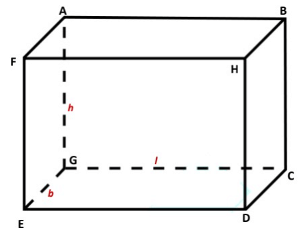The lateral surface area of a cuboid
= Area of face HBCD + Area of face ABCG + Area of face GEFA + Area of face FHDE.
= (b × h) + (l × h) + (b × h) + (l × h).
= 2(bh + lh).
= 2h (b + l).
Lateral surface area of a cuboid = 2h (b + l).

Example: Find the lateral surface area of a wooden box whose length, breadth and height are 10 cm, 8 cm, and 6 cm, respectively.

Clearly, this wooden box is in the form of a cuboid.
Here, l = 10 cm, b = 8 cm and h = 6 cm.
Therefore, lateral surface area of the wooden box = 2h (b + l).
= 2 × 6 cm(8 cm +10 cm) = 2× 6 cm(18 cm) = 12 cm × 18cm
= 216 cm2
Hence, the lateral surface area of the wooden box = 216 cm2

### Surface Area of a Cube

Cube: In our everyday life, we come across objects like a dice, Rubik’s cube, Sugar cube, and Ice cube, etc. These objects are in the shape of a cube. All these objects are made of six square plane regions.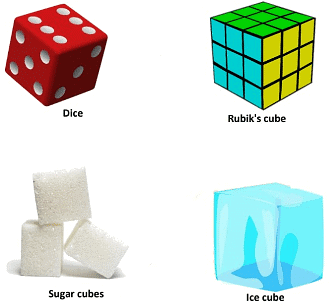A cube is a three-dimensional shape whose length, breadth and height are all equal. The cube has six surfaces called faces. Each face of a cube is a square, two adjacent faces of a cube meet in a line segment called edge and all of a cube's corners (called vertices) are right angles. Ultimately, a cube has the shape of a square box.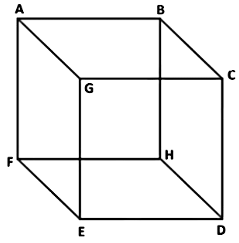A cube has six faces (FHDE, FAGE, ABCG BCHD, ABFH & GCDE), eight vertices (A, B, C, D, E, F, G & H), twelve edges (AB, BC, CG, GA, BH, HD, DC, DE, FE, FH, AF, & GE).
In the case of a cube, its length, breadth, and height are equal.
Then, side of cube = Length = Breadth = Height
So, the figure with these dimensions would be like the shape shown below. Here, side of cube = a.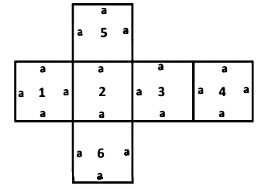So, the sum of the areas of the six squares is:
Area of square 1 = (a × a) + Area of square 2 = (a × a) + Area of square 3 = (a × a) + Area of square 4 = (a × a) + Area of square 5
= (a × a) + Area of square 6 = (a × a).
⇒ Surface Area of Cube = 2(a × a) + 2(a × a) + 2(a × a).
= 2[(a × a) + (a × a) + (a × a)].
= 2(a2 + a2 + a2).
= 2(3a2).
= 6 a2.
Where a is the length of edges of the cube.
Surface Area of Cube = 6 a2

Example: Find the surface area of a Rubik’s cube whose edge is 6cm.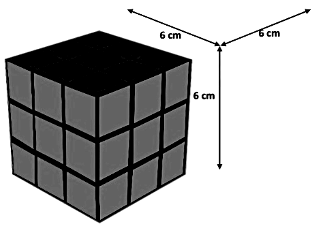Therefore, the surface area of Rubik’s cube = 6a2.
= 6(62)cm2.
= 6 × 36 cm2.
= 216 cm2.
Hence, the surface area of Rubik's cube = 216 cm2.

Lateral Surface Area of a Cube: If out of the six faces of a cube, we only find the sum of the areas of four faces leaving the bottom and top faces. This sum is called the lateral surface area of the cube.
Consider a cube of the side as ‘a’ which is shown in the figure below.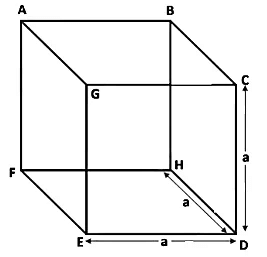Lateral surface area of cube
= Area of face HBCD + Area of face CDEG + Area of face GEFA + Area of face ABHF.
= (a × a) + (a × a) + (a × a) + (a × a).
= a2 + a2+ a+ a2= 4a2
Lateral surface area of a cube = 4a2

Example 1: Find the lateral surface area of a dice whose edge is 5cm.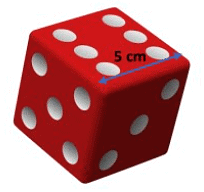Clearly, dice is in the form of a cube.
Here, length of edge of dice = a = 5 cm.
Therefore, Lateral surface area of the dice = 4a2.
= 4(52)cm2.
= 4 × 25 cm2.
= 100 cm2.
Hence, the lateral surface area of the dice = 100 cm2.

Example 2:Five cubes each of side 5 cm are joined end to end. Find the surface area of the resulting cuboid.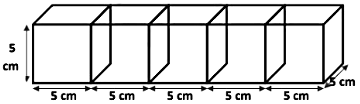The dimensions of the cuboid so formed are as under :
l = length = (5 + 5 + 5 + 5 + 5) cm = 25 cm,
b = breadth = 5 cm and h = height = 5 cm.
So, surface area of the cuboid = 2(lb + bh + lh).
= 2(25 cm × 5 cm + 5 cm × 5 cm + 25 cm × 5 cm).
= 2(125 cm2 + 25 cm2 + 125 cm2).
= 2(275 cm2).
= 550 cm2.
Hence, Surface area of the cuboid formed = 550 cm2.

### Surface Area of a Right Circular Cylinder

Right Circular Cylinder: In our everyday life, we come across objects like a battery, a soda cane, a candle, chalk, a gas cylinder, a roll of paper towels, a garden roller, circular pillars, and circular pipes, etc. All these solids have a curved (lateral) surface with circular ends with an equal radius. Such objects are in the shape of right circular cylinders.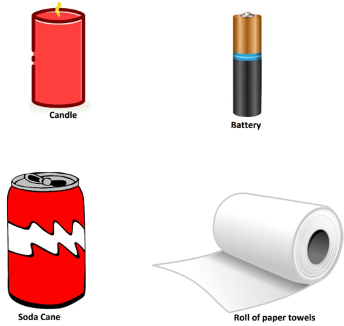A right circular cylinder has two plane ends. Each plane end is circular, and the two plane ends are parallel. Each of the plane ends is called a base of the cylinder.
The line segment joining the centres of these two planes is called the axis of the cylinder. When this axis is not perpendicular to the circular ends, then the cylinder is not a right circular cylinder.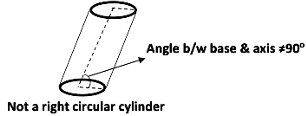If we take several circular sheets and stack them up vertically as shown in the below figure, then we get a right circular cylinder.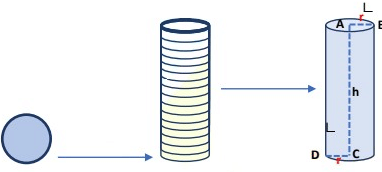Base: Each of the circular ends of the cylinder is called its base.
So, CD and AB are the radius of the cylinder. And both are equal to each other and the length of each circular edge is 2πr.
Height: The shortest distance between two circular bases in a cylinder is called the height of the cylinder. So, AC is the height of the cylinder. AC = h.
If we take a rectangular sheet which has breadth equal to the height of the cylinder, then place the edge of the sheet of paper along with the height of the cylinder and hold it fast. Now, wrap the sheet around the cylinder then cut- off the sheet along with the height.
Remove the piece of the sheet so cut-off and spread it on a plane surface. We will find that sheet is a rectangle of length 2πr (equal to the length of each circular edge) and breadth h as shown in the below figure.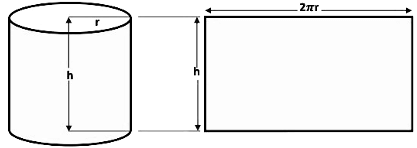The area of the sheet gives us the curved surface area of the cylinder.
So, the curved surface area of the cylinder = area of the rectangular sheet
= perimeter of the base of the cylinder × h.
= 2πr × h.
where r is the radius of the base of the cylinder and h is the height of the cylinder.
Curved Surface Area of the Cylinder = 2πrh
If the top and bottom of the cylinder are also to be covered, then we need two circles to do that, each of radius r, and thus having an area of π2  each, giving us the total surface area as 2πrh + πr2+ πr2 .
Therefore, the total surface area of the cylinder = 2πrh + 2πr2.
= 2πr (h + r).
Where h is the height of the cylinder and r is its radius.
Total Surface Area of the Cylinder = 2πr (h + r).

Example 1: The curved surface area of a soda can of height 7 cm is 44 cm2. Find the radius of the base of the soda can.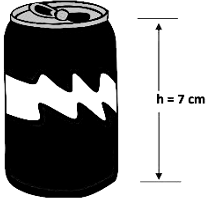Clearly, soda can is in the form of a right circular cylinder.
Here, Curved surface area of the soda can and its height is 44 cm2 and 7 cm respectively.
We know that,
Curved Surface Area of Cylinder = 2πrh.
So, Curved Surface Area of the soda cane = 2πrh.
44 cm2 = 2 × 22/7 × r × 7 cm.
⇒ 44 cm2 = 2 × 22 × r cm.
⇒ 44 cm2 = 44r cm.
⇒ r = 44cm2 / 44cm
⇒ r = 1 cm.
Hence, the radius of the base of the soda cane = 1 cm.

Example 2: Find the total surface area of a candle if the height and diameter of the base of the candle are 6.5 cm and 1 cm respectively.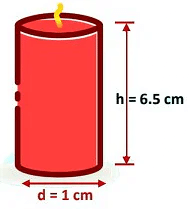The candle is in the form of a right circular cylinder.
Here, the diameter of the base of the candle and its height is 1 cm and 6.5 cm respectively.
⇒ Radius of the base of the candle = d/2 =  1cm/2 = 0.5 cm.
We know that,
Total Surface Area of the cylinder =2πr (h + r).
So, the total Surface Area of the candle =2πr (h + r).
= 2  ×  22/7 × 0.5 cm (6.5 cm + 0.5 cm).
= 2 × 22/7 × 1/2 cm (7 cm).
= 22 cm2.
Here, the total Surface Area of the candle = 22 cm2.

### Surface Area of Sphere

Sphere: In our everyday life, we come across objects like the earth, a world globe, a soccer ball, a basketball, the moon, etc. These are a three-dimensional geometrical object which is in the shape of a sphere.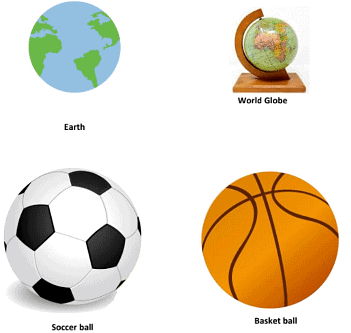If we take a circle as we know that it is a plane closed figure whose every point lies at a constant distance (called radius) from a fixed point, which is called the center of the circle. Now if we paste a long string along a diameter of a circular disc. Hold the string with your own hands on either side of the disc and rotate the disc about the string a number of times. We recognize the shape that the circular disc is formatting as it rotates around the string, then it reminds us of a shape as shown in the below figure and we get a  sphere.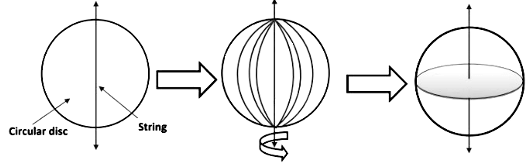When a circle forms a sphere on rotation, it becomes the center of the sphere. So, a sphere is a three-dimensional figure which is made up of all points in the space, which lie at a constant distance called the radius, from a fixed point called the center of the sphere. If on a sheet of paper, we draw four circles with a radius equal to the radius of the sphere as shown in the figure below.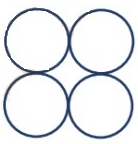Then start filling the circles one by one, with the string we had wound around the sphere. The string, which had completely covered the surface area of the sphere, has been used to completely fill the regions of four circles, all of the same radii as of the sphere. This suggests that the surface area of a sphere of radius
r = 4 times the area of the circle of radius r
= 4 × πr= 4πr2 .
Surface Area of Sphere = 4πr2
Where r is the radius of the sphere.
Let us take a sphere, and slice it exactly through the middle with a plane that passes through its center. It gets divided into two equal parts. It is called Hemisphere.
(Because 'hemi' also means 'half') Hemisphere: It has two faces one is a curved face and the other is a flat face (base).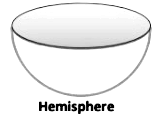The curved surface area of a hemisphere is half the surface area of the sphere,
which is 1/2  ×4πr2 .
Therefore, Curved Surface Area of a Hemisphere = 2πr2 .
Where r is the radius of the sphere of which the hemisphere is a part.
Now taking the two faces of a hemisphere, its total surface area is 2πr2 + πr2.
So, Total surface area of a hemisphere = 3 πr2.

Example 1: Find the surface area of a tennis ball of radius 14 cm.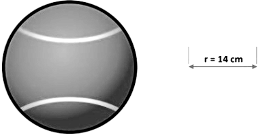Clearly, the tennis ball is in the form of a sphere.
Here, the radius of the sphere is 14 cm.
We know that,
Surface Area of the sphere =4πr2.
Therefore, Surface Area of tennis ball =4πr2.
= 4 × 22/7 × (14 cm)2.
= 4 × 22/7 × 14cm × 14 cm.
= 4 × 22 × 2cm × 14 cm.
= 2464 cm2.
Hence, Surface area of the tennis ball is 2464 cm2.

Example 2: Find the curved surface area and the total surface area of the half slice of watermelon of radius 7 cm.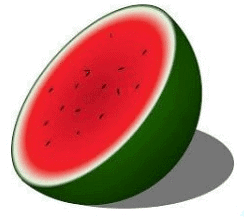Clearly, half slice of watermelon is in the form of a hemisphere.
Here, radius of hemisphere is 7 cm.
We know that,
Curved surface area of the hemisphere =2πr2.
Therefore, Curved surface Area of half slice of watermelon =2πr2.
= 2 × 22/7  × (7 cm)2.
= 2 × 22/7 × 7cm × 7 cm.
= 2 × 22 × 1cm × 7 cm.
= 308 cm2.
Hence, Curved Surface area of half slice of watermelon is 308 cm2.
Also, we know that
The total surface area of hemisphere = 3πr2.
So, the total surface area of half slice of watermelon = 3πr2.
= 3 ×227× (7 cm)2.
= 3  × 22/7 × 7cm × 7 cm
= 3 × 22 × 1cm × 7 cm.
= 462 cm2.
Hence, the total surface area of half slice of watermelon = 462 cm2.

### Volume of a Cuboid and a Cube

Volume of a solid object is the measure of the space occupied by it.

If the object is hollow, then its interior is empty, which can be filled with either air or some liquid that will take the shape of the hollow object. The volume of the substance that can fill the interior of the hollow object is called it's capacity.
In other words, the volume of an object is the measure of the space it occupies, and the capacity of a hollow object is the volume of substance its interior can accommodate.

Volume of a cuboid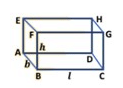When we talk about the volume of a cuboid, we mean the measure of the space occupied by the cuboid.
Let there be a cuboid of length l, breadth b and height h.
The area of the rectangular base ABCD of the cuboid is (l × b). If we take rectangular sheets congruent to the base ABCD of the cuboid and the sheets are put one over the other as shown in the figure. Then, the height to which the sheets are stacked to form the cuboid is h.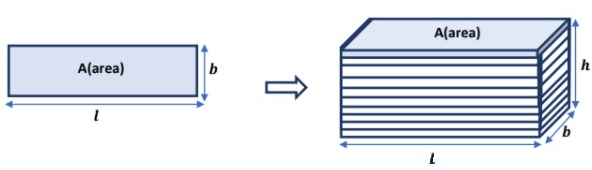The measure of the space occupied by the cuboid = the area of the rectangular sheet × height.
= A × h = V. where A and V are respectively area and volume of a cuboid.
That is, the Volume of Cuboid = base area × height
= length × breadth × height.
= l × b × h.
Volume of Cuboid = base area × height = length × breadth × height.
Where l, b and h are respectively the length, breadth, and height of the cuboid.
Volume of a Cube
We know that a cube is a special type of cuboid whose length, breadth and height are all equal i.e. length = breadth = height.
So, the volume of a cube of side a is given by
V = a × a × a = a3 = (side)3 .
Where V and a are respectively the volume and side of a cube.

Example 1: A matchbox measure 4 cm × 2 cm × 1 cm. What will be the volume of a packet containing 15 such boxes?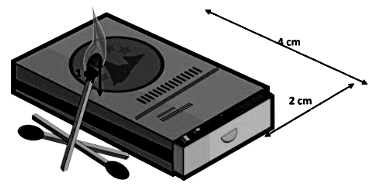The matchbox is in the form of a Cuboid. So, first, we will find the volume of one matchbox. Then, we will find the volume of the packet containing 15 such matchboxes.
Here, length, breadth, and height of matchbox are 4 cm, 2 cm, and 1 cm respectively.
Volume of Cuboid = length × breadth × height = l × b × h.
Therefore, the volume of 1 matchbox = l × b × h
= 4 cm × 2 cm × 1 cm.
= 8 cm3.
The volume of 15 matchboxes = 15 × Volume of 1 matchbox Hence, volume of 15 matchboxes
= 15 × 8 cm3 = 120 cm3.

### The volume of a Right Circular Cylinder

Suppose that we take circular sheets of radius r and stack up vertically as shown in the figure below to form a right circular cylinder of height h.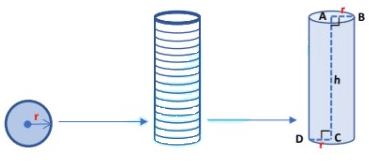Therefore, Volume of the cylinder = Measure of the space occupied by the cylinder.
The volume of Cylinder = The area of each circular sheet × height.
The volume of right circular Cylinder = Area of the base × height.
= πr2 × h
= πr2h.
Volume of a right circular Cylinder = πr2h.
Where r and h are the radius of the base and height of the right circular cylinder respectively.

### Volume of a hollow Cylinder

Suppose that R and r be the external and internal radii of a hollow cylinder (shaded in blue) & h be its height as shown in the figure below.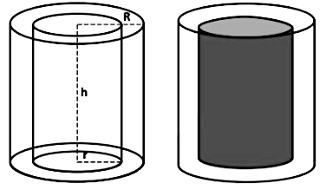The volume of the hollow cylinder = Exterior volume – Interior volume
= πR2h − πr2h
= πh (R2 − r2)
The volume of the hollow cylinder = πh (R− r2)
Where h is the height of the hollow cylinder.

Example 1: Find the volume of a soda can, if the radius of its base and height is 7 cm and 18 cm respectively.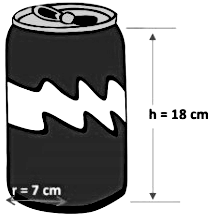Clearly, soda cane is in the form of a right circular cylinder.
Suppose that, height and radius of soda cane are 18 cm and 7 cm respectively.
Volume of a cylinder = πr2h.
So, Volume of the soda can = πr2h.
= 22/7 × (7 cm)2 × 18 cm.
= 22/7 × 7 cm × 7 cm × 18 cm.
= 22 × 1 cm × 7 cm × 18 cm.
= 2772 cm3.
Hence, the volume of a soda can is = 2772 cm3.

Example 2: The thickness of a hollow wooden cylinder is 2 cm. It is 35 cm long and its inner radius is 5 cm. Find the volume of the wood required to make the cylinder.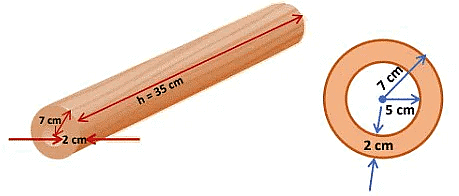We have,
r = radius of the inner cylinder = 5 cm.
The thickness of the cylinder = 2 cm.
∴ R = Outer radius of the cylinder = (5 cm + 2 cm) = 7 cm.
h = height of the cylinder = 35 cm.
∴ The volume of the wood = πh (R2 − r2).
= 22/7 × 35 cm [(7 cm)− (5 cm)2]
= 22/7 × 35 cm [(7 cm)2 − (5 cm)2].
= 22/7 × 35 cm [(7 cm + 5 cm) × (7 cm – 5 cm)].
= 22/7 × 35 cm × 12 cm × 2 cm.
= 22 × 5 cm × 12 cm × 2 cm.
= 2640 cm3.
Hence, the volume of the wood is 2640 cm3.

### Volume of a right circular cone

To find the volume of a right circular cone, let us consider the following experiment.

We take a conical cup of radius r and height h. Also, take a cylindrical jar of radius r and height h.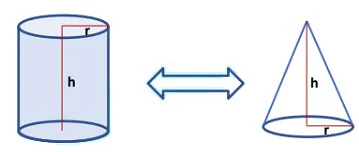Fill the conical cup with water to the brim and transfer the water to the cylindrical jar. Repeat the process two times more. We will find that 3 conical cups will fill the cylindrical jar completely.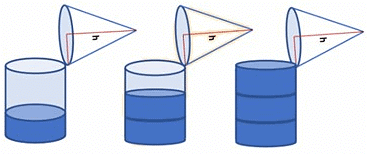So, we conclude that 3(Volume of a cone of radius r and height h) = (Volume of a cylinder of radius r and height h) = πr2h cubic units.
∴ The volume of a cone of radius r and height h = 1/3 πr2h cubic units.
Thus, Volume of a cone = 1/3 πr2h
= 1/3 (Area of the base) × (height).

Example: Find the volume of a waffle cone 18 cm high, if the radius of its base is 7 cm.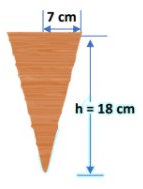The waffle cone is in the form of a right circular cone.
Suppose, the height and the radius of the waffle cone are 18 cm and 7 cm respectively.
We know that,
Volume of a cone = 1/3 πr2h.
So, Volume of a waffle cone = 13πr2h.
= 1/3 × 22/7 × (7 cm)2 × 18 cm.
= 1/3 × 22/7 × 7 cm × 7 cm × 18 cm.
= 22 × 1 cm × 7 cm × 6 cm.
= 924 cm3.
Hence, the volume of the waffle cone = 924 cm3

### Volume of a Sphere

To find the volume of a sphere, let us consider the following experiment.

We take two spheres of different radii, and a container big enough to be able to put each of the spheres into it, one at a time. Also, take a large trough in which we can place the container. Then, fill the container up to the brim with water as shown in figure (1) below. Now, carefully place one of the spheres in the container. Some of the water from the container will overflow into the trough in which it is kept as shown in figure (2) below.
Carefully pour out the water from the trough into a measuring cylinder (graduated cylindrical jar) an measure the water overflowed as shown in figure (3).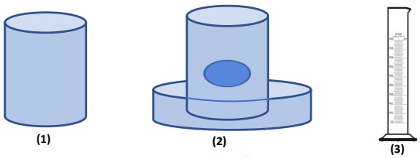Suppose the radius of the immersed sphere is r, then the volume of overflow water is equal to 4/3πr3.
Once again repeat the procedure done just now, with a different size of the sphere. Find the radius R of this sphere and then calculate 4/3πr3
Once again, this value is nearly equal to the measure of the volume  of the water displaced (overflowed) by the sphere.
Therefore, the volume of the sphere is equal to 4/3π times the cube of its radius. This gives us the idea that Volume of a sphere = 4/3πr3.
Where r is the radius of the sphere.
Since a hemisphere is half of a sphere, then
Volume of a hemisphere = 4/3 πr3.
Where r is the radius of the sphere.

Example 1: Find the volume of a football of radius 7 cm.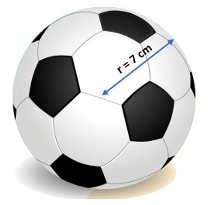Clearly, football is in the form of a sphere which radius is 7 cm.
We know that,
Volume of a sphere =4/3 πr3.
So, Volume of the football = 4/3}πr3.
=  4/3 × 22/7 × (7 cm)3.
= 4/3 × 22/7 × 7 cm × 7 cm × 7 cm.
=  4/3  × 22 × 1 cm × 7 cm × 7 cm.
= 1437.33 cm3.
Hence, volume of a football is = 1437.33 cm3.

Example 2: Find the volume of a bowl of radius 7 cm.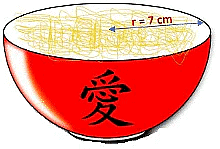The bowl is in the shape of a hemisphere. Hemisphere has a radius of 7 cm.
We know that,
Volume of a hemisphere = 2/3 πr3.
So, Volume of a hemisphere = 2/3 πr3.
= 2/3  × 22/7 × (7 cm)3.
= 2/3× 22/7 × 7 cm × 7 cm × 7 cm.
= 2/3 × 22 × 1 cm × 7 cm × 7 cm.
= 718.66 cm3.
Hence, the volume of a hemisphere is 718.66 cm3.

The document Surface Area & Volumes Chapter Notes | Mathematics (Maths) Class 9 is a part of the Class 9 Course Mathematics (Maths) Class 9.
All you need of Class 9 at this link: Class 9

## FAQs on Surface Area & Volumes Chapter Notes - Mathematics (Maths) Class 9

 1. What is surface area?Ans. Surface area is the total area of all the surfaces of a three-dimensional object. It is measured in square units.
 2. How is surface area different from volume?Ans. Surface area refers to the total area of the outer surfaces of a three-dimensional object, while volume refers to the amount of space occupied by the object.
 3. How is the surface area of a cube calculated?Ans. The surface area of a cube can be calculated by multiplying the length of one side by itself, and then multiplying the result by 6 (since a cube has 6 equal sides).
 4. What is the formula for finding the surface area of a cylinder?Ans. The formula for finding the surface area of a cylinder is 2πr(r+h), where r is the radius of the base and h is the height of the cylinder.
 5. How can the surface area of a sphere be calculated?Ans. The surface area of a sphere can be calculated using the formula 4πr², where r is the radius of the sphere.

## Mathematics (Maths) Class 9

62 videos|438 docs|102 tests

## Mathematics (Maths) Class 9

62 videos|438 docs|102 tests
Signup to see your scores go up within 7 days! Learn & Practice with 1000+ FREE Notes, Videos & Tests.
10M+ students study on EduRev
Track your progress, build streaks, highlight & save important lessons and more!(Scan QR code)
Related Searches

,

,

,

,

,

,

,

,

,

,

,

,

,

,

,

,

,

,

,

,

,

;